# 基于VISSIM仿真的高速公路事故管控策略研究Research on Expressway Accident Management and Control Strategy Based on VISSIM Simulation

• 全文下载: PDF(765KB)    PP.390-396   DOI: 10.12677/OJTT.2018.76047
• 下载量: 247  浏览量: 664   科研立项经费支持

In order to reduce traffic congestion quickly and accurately after traffic accidents on express ways, in this paper, we use toll station data to establish freeway vehicle speed model. According to the speed threshold, we can judge whether there is a traffic accident on a certain road. According to the duration of accidents, a decision-making method of management and control based on VISSIM simulation is proposed. The “queue length” parameter is selected as the evaluation index of the management and control scheme, and the prohibition time and the number of entrances at the charging stations upstream of the accident are controlled. Taking a typical expressway section in Shandong Province as an example, the results of VISSIM simulation and practical application show that: the use of vehicle speed model and the implementation of management and control scheme have a scientific assistant decision-making role, and provide a strong technical support for the real-time management and control of expressway.

1. 引言

2. 高速公路管控模型

2.1. 路段模型

2.2. 速度模型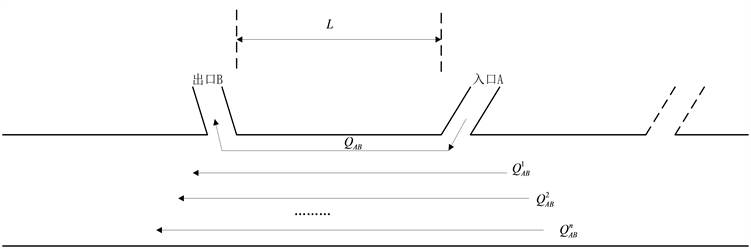Figure 1. Section model

1) 根据前面条件设定，设待求AB区间的区间平均速度为 ${\stackrel{¯}{V}}_{AB}$ ，其计算公式如下：

${\stackrel{¯}{V}}_{AB}={\alpha }_{1}{\stackrel{¯}{v}}_{AB}+{\alpha }_{2}{\stackrel{¯}{v}}_{AB}^{\text{'}}$ (1)

${\stackrel{¯}{v}}_{AB}$ 的计算方法如下：

${\stackrel{¯}{v}}_{AB}=\frac{L}{\frac{1}{N}\underset{i}{\overset{N}{\sum }}\left({t}_{i出}-{t}_{i入}\right)}$ (2)

${\stackrel{¯}{v}}_{AB}^{\text{'}}$ 的计算方法如下：

${\stackrel{¯}{v}}_{AB}^{\text{'}}=\frac{1}{k}\underset{k=1}{\overset{1}{\sum }}\left(\frac{{L}_{k}}{\frac{1}{{M}_{k}}\underset{j}{\overset{{M}_{k}}{\sum }}\left({t}_{j出}-{t}_{j入}\right)}\right)$ (3)

2.3. 收费站入口控制模型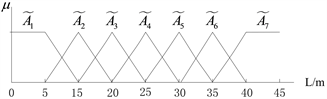Figure 2. Membership function of event severity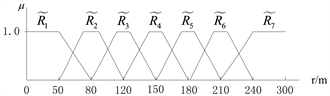Figure 3. Membership function of regional influence range

$\left\{VS,S,M,L,VL\right\}$ ，其相应隶属度值如表1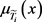表示各模糊子集值在等级X中对应的隶属度值。Table 1. The corresponding membership values of each fuzzy subset value in rank X

${R}_{p}$ ：IF $\stackrel{˜}{A}$ is $\stackrel{˜}{{A}_{i}}$ THEN $\stackrel{˜}{R}$ is $\stackrel{˜}{{R}_{j}}$ AND $\stackrel{˜}{T}$ is $\stackrel{˜}{{T}_{k}}$

$\underset{˜}{U}=\underset{˜}{A}\cdot \underset{˜}{R}$ (4)

${u}^{*}=\frac{\underset{i=1}{\overset{n}{\sum }}\mu {\underset{˜}{U}}_{1}\left({u}_{i}\right){u}_{i}}{\underset{i=1}{\overset{n}{\sum }}\mu {\underset{˜}{U}}_{1}\left({u}_{i}\right)}$ (5)

3. 交通事故参数设置及仿真

3.1. 仿真参数设置

3.2. 排队长度原始数据的获取

VISSIM的排队长度输出文件里记录了平均排队长度、最大排队长度、停车次数，表2列出了事故持续20分钟不实施管控方案时仿真的部分输出数据。Table 2. Data table of “queue length” part

Continued

4. 仿真方案对比分析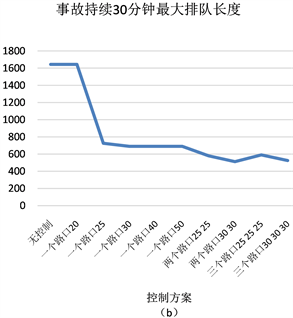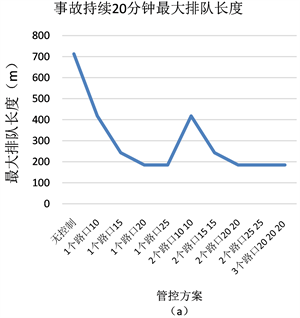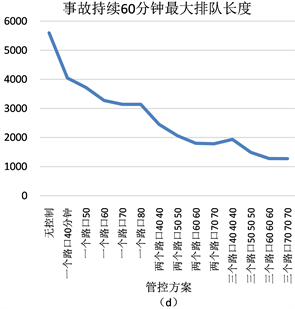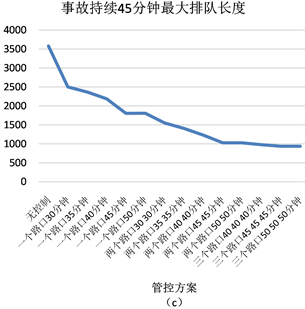Figure 4. Comparison of control plans under different accident durationTable 3. Best management and control plan

5. 结论

  陈昊, 陆建. 基于VISSIM仿真的高速公路事故交通影响[J]. 长安大学学报(自然科学版), 2015, 35(S1): 227-229.  Transportation Research Board (2000) Highway Capacity Manual. National Research Council, Washington DC.  秦焕美, 曹静. 交通规划与仿真软件实验指导书[M]. 北京: 北京工业大学出版社, 2014.  李伟楠, 熊军. 基于VISSIM 仿真的城市快速路设计方案论证及对比分析[J]. 华东公路, 2016(2): 55-57.  Zhu, Z., Chen, S, Yang, Y., Hu, A. and Zheng, X. (2016) VISSIM Simulation Based Expressway Exit Control Modes Research. Procedia Engineering, 137, 738-746. https://doi.org/10.1016/j.proeng.2016.01.311  Yang, Y.Q., Hu, A.X., Chen, S.H. and Zheng, X.Y. (2015) Study on Off-Ramp Metering of Expressways Based on VISSIM Simulation. Road Traffic & Safety, 15, 8-15.